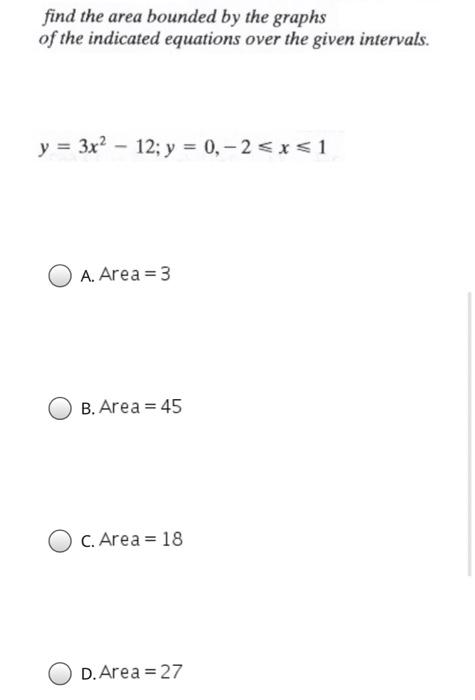### Create an Account

Already have account?

### Forgot Your Password ?

Home / Questions / find the area bounded by the graphs of the indicated equations over the given intervals. y...

# find the area bounded by the graphs of the indicated equations over the given intervals. y = 3x2 – 12; y = 0, -2

find the area bounded by the graphs of the indicated equations over the given intervals. y = 3x2 – 12; y = 0, -2May 06 2021 View more View Less

#### Answer (Solved)Subscribe To Get Solution